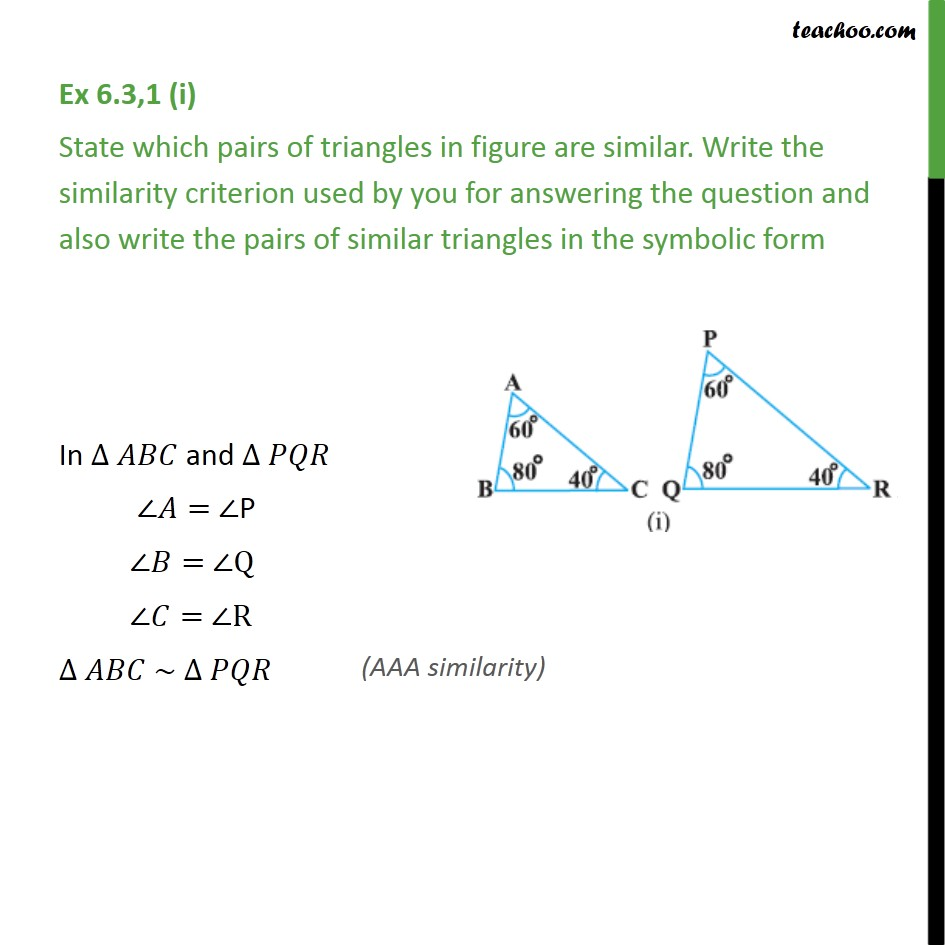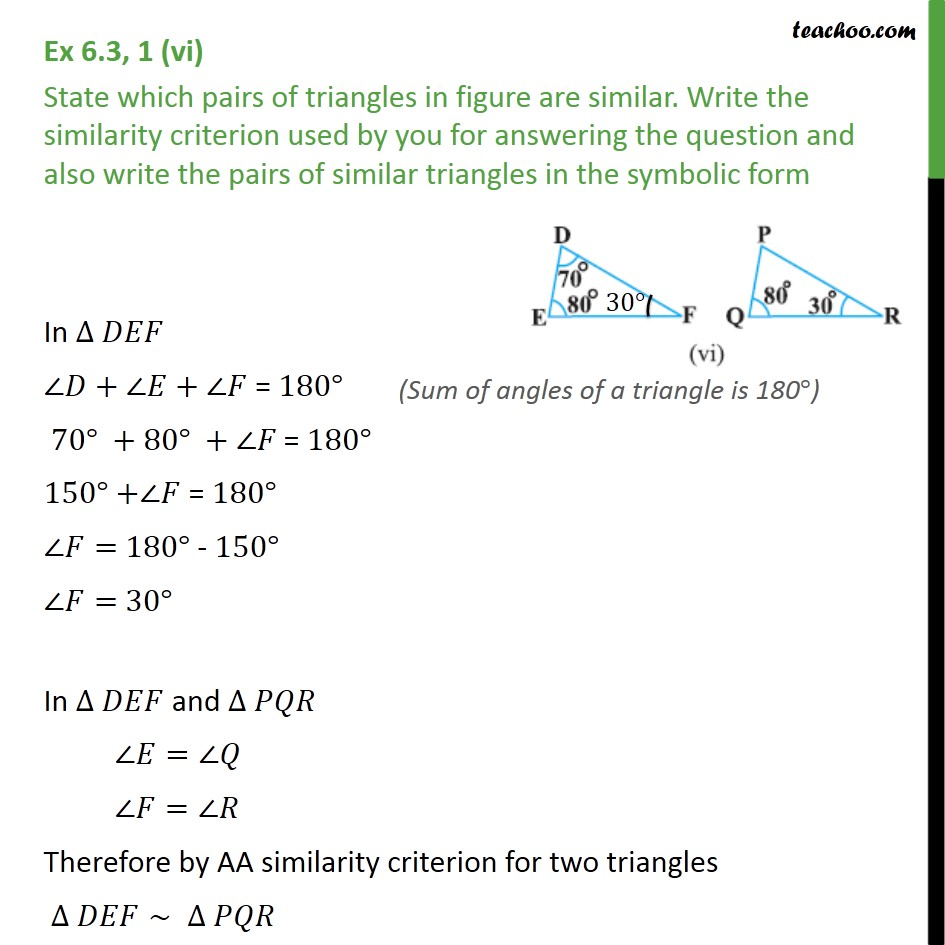1. Chapter 6 Class 10 Triangles
2. Concept wise
3. AA Similarity

Transcript

Ex 6.3,1 (i) State which pairs of triangles in figure are similar. Write the similarity criterion used by you for answering the question and also write the pairs of similar triangles in the symbolic form In ∆ 𝐴𝐵𝐶 and ∆ 𝑃𝑄𝑅 ∠𝐴= ∠P ∠𝐵= ∠Q ∠𝐶= ∠R ∆ 𝐴𝐵𝐶 ∼ ∆ 𝑃𝑄𝑅 Ex 6.3, 1 (vi) State which pairs of triangles in figure are similar. Write the similarity criterion used by you for answering the question and also write the pairs of similar triangles in the symbolic form In ∆ 𝐷𝐸𝐹 ∠𝐷+∠𝐸+∠𝐹 = 180° 70°" "+80°" "+∠𝐹" = " 180° 150° +∠𝐹 = 180° ∠𝐹= 180° - 150° ∠𝐹= 30° In ∆ 𝐷𝐸𝐹 and ∆ 𝑃𝑄𝑅 ∠𝐸=∠𝑄 ∠𝐹=∠𝑅 Therefore by AA similarity criterion for two triangles ∆ 𝐷𝐸𝐹∼" " ∆ 𝑃𝑄𝑅

AA Similarity